# Triangle Calculation: Find C

Triangle Calculation: Find C

## Preface

Learn about triangle calculation to find H, triangle area calculator and right angled triangle calculation in the concept of triangle calculation to find C. Check out the interactive simulations to know more about the lesson and try your hand at solving a few interesting practice questions at the end of the page.

Become a champ of triangle calculation to find C in just 4 minutes!

## Introduction

The Law of Sines was provided by Persian mathematicians in the 10th century.

 1 What is "C" in Triangle Calculation? 2 What Are the General Formulae to Calculate "C" in a Triangle? 3 Tips and Tricks 4 How to Calculate the Value of "C" in Triangle? 5 Solved Examples on Triangle Calculation 6 Practice Questions on Triangle Calculation 7 Frequently Asked Questions (FAQs)

Math represents ideas, creative-thinking, and problem-solving. We, at Cuemath, understand this and bridge creative thinking with numbers. At its core, mathematics is simple. And we are good at identifying simplicity.

and experience Cuemath’s LIVE online class with your child.

## What is "C" in Triangle Calculation?

### General case

"C" generally represents the third side in a triangle.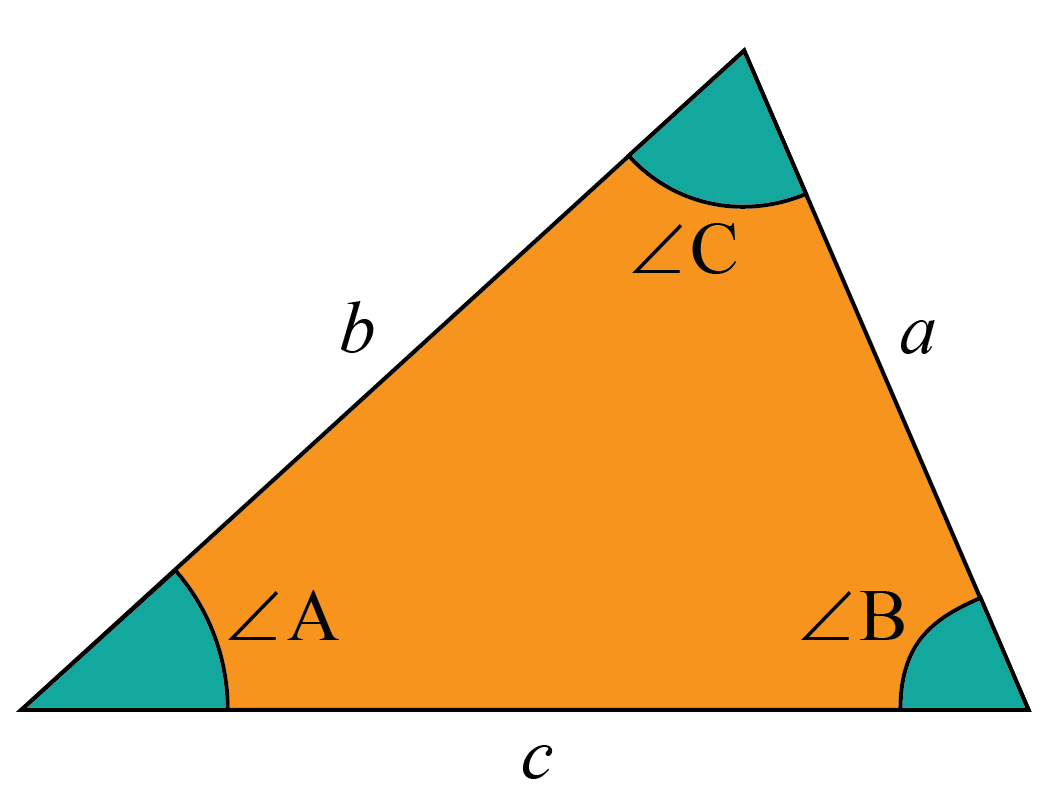### Right-angled triangle

In case of a right-angled triangle, it is referred to as "c" or the hypotenuse (h).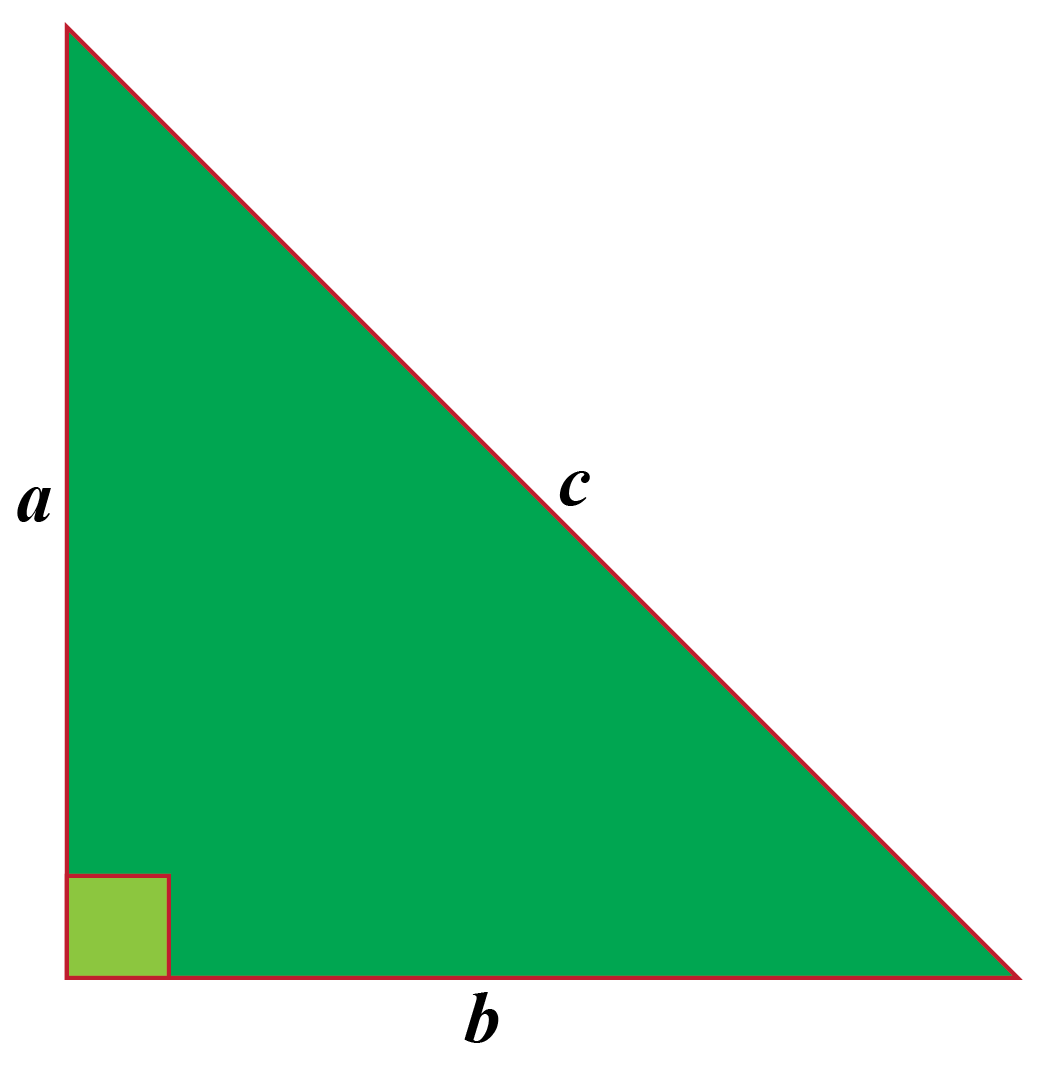## What Are the General Formulas to Calculate "C" in a Triangle?

### General case

To calculate "c" in any triangle we use sine and cosine formulas given as below:

Law of sines:

The Law of Sines is given by the following formula:

 $$\dfrac{a}{\sin A} = \dfrac{b}{\sin B} = \dfrac{c}{\sin C}$$

It is given as the ratio of side to the sine of the opposite angle.

Law of cosines:

The Law of Cosines is given by the following formula:

 $$\cos A = \dfrac{b^2+c^2-a^2}{2bc}$$ $$\cos B = \dfrac{a^2+c^2-b^2}{2ac}$$ $$\cos C = \dfrac{a^2+b^2-c^2}{2ab}$$

It is given as the ratio of subtraction of length of opposite side squared from the  sum of the lengths of  adjacted sides squared to the product of two times the lengths of adjacent sides.

To find the area of triangle having sides A,B and C, we use Heron's formula.

 Perimeter of triangle = ( A+B+C ) $$S = \dfrac{A+B+C}{2}$$ $$Area = \sqrt{S(S-A)(S-B)(S-C)}$$

Let's try to apply our learning on Heron's formula using the below given simulation.

### Special Case: Right-angled triangle

In case of right angled triangle, the side "c" (or hypotenuse) is calculated using the Pythagorean Theorem. According to the theorem, the sum of the squares of base and perpendicular is always equal to the square of the hypotenuse.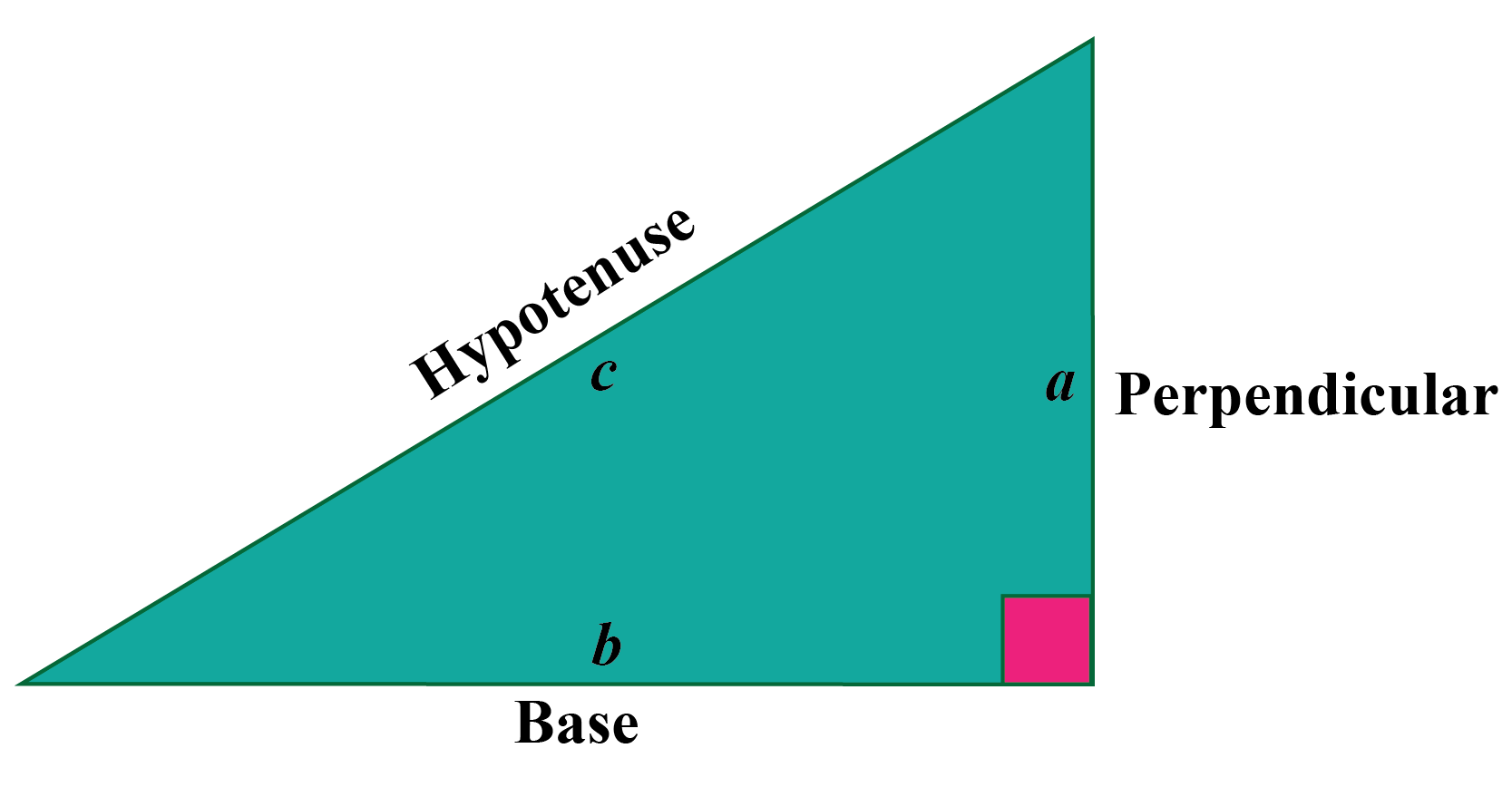It is given by the following formula:

 $$a^2 + b^2 = c^2$$

Area of triangle as per the above figure will be given by formula,

 A = $$\dfrac{1}{2} \times b \times a$$

Try your hand at the simulation to find the area of triangle.Tips and Tricks

• In the case of right-angled triangle, if the Law of Sines is applied, sin C = sin 90= 1 . Hence the Law of Sines can be written as $$\dfrac{a}{\sin A} = \dfrac{b}{\sin B} = c$$

Want to understand the “Why” behind the “What”? Explore triangle calculation:find c with our Math Experts in Cuemath’s LIVE, personalized and interactive online classes.

Make your kid a Math Expert. Book a FREE trial class today!

## How to Calculate Value of "C" in a Triangle?

Let's learn how to calculate value of c in a triangle.

### Example 1

Claire drew a triangle with 2 sides and 3 angles as given below. Help her do the triangle calculation to find C and angles A and C.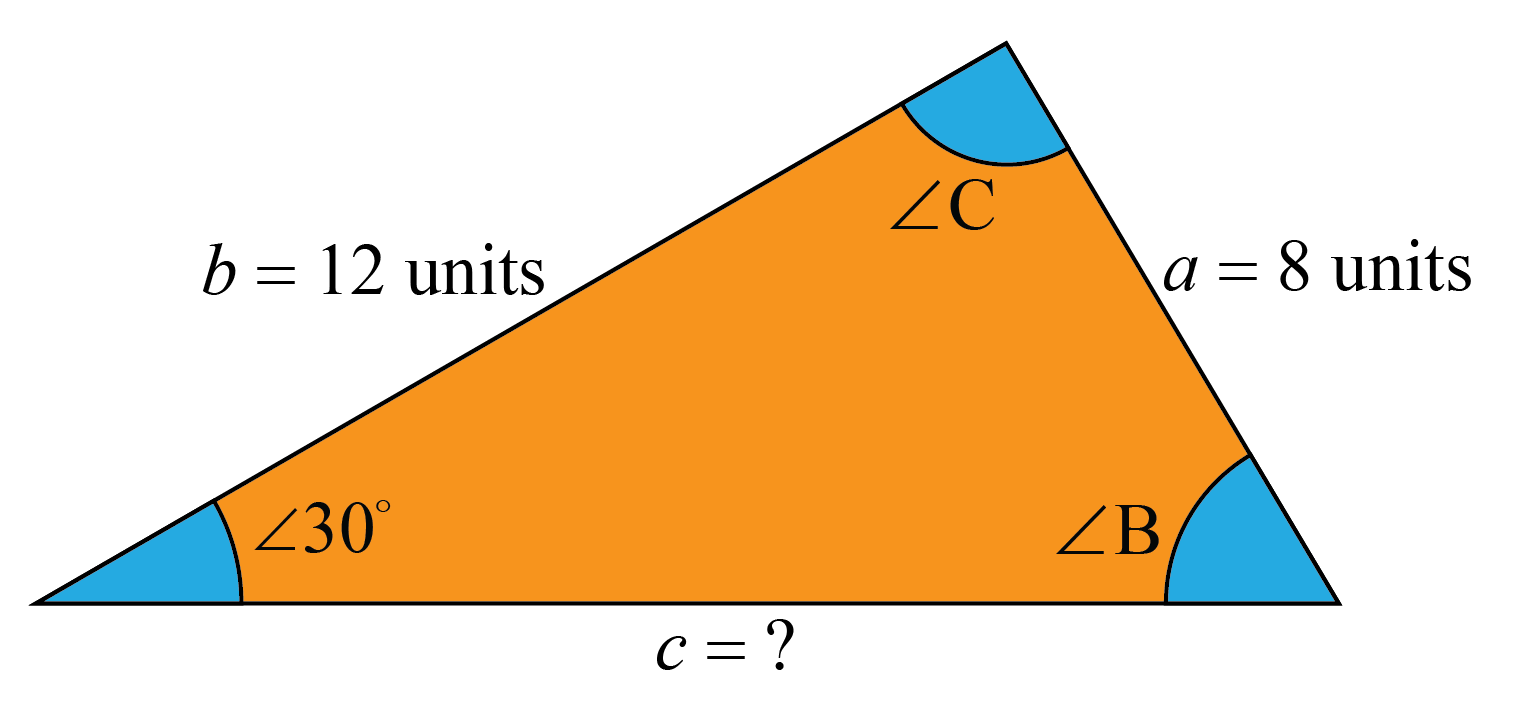To find the third side, Claire uses the Law of Sines which is given as follows:

$$\dfrac{a}{\sin A} = \dfrac{b}{\sin B} = \dfrac{c}{\sin C}$$

Given the value of sides a and b, 8 units and 12 units respectively and A = 30. On applying the Law of Sines she gets,

$$\dfrac{8}{\sin 30}$$= $$\dfrac{12}{\sin B}$$

Hence, $$\sin B$$ = $$\dfrac{12 \times \sin 30}{8}$$

$$\sin B = \dfrac{6}{8}$$

sin 30 = $$\dfrac{1}{2}$$, as refererred from trigonometric values.

B = $$\sin^{ - 1} 0.75$$

B =  48.6∘

Sum of all angles in a triangle is 180

Hence, A + B + C = 180∘

30 + 48.6 + C = 180∘

C = 180∘ - (30 + 48.6) = 101.4∘

Hence, by the Law of Sines,

$$\dfrac{12}{\sin 48.6} = \dfrac{c}{\sin 101.4}$$

c = $$\dfrac{12 \times \sin 101.4}{\sin 48.6}$$

c = 15.8 units

### Example 2

Isabella took an example of a right-angled triangle. The area of triangle was 30 sq.unit having length of base equal to 5 units. Help Isabella do the triangle calculation to find H.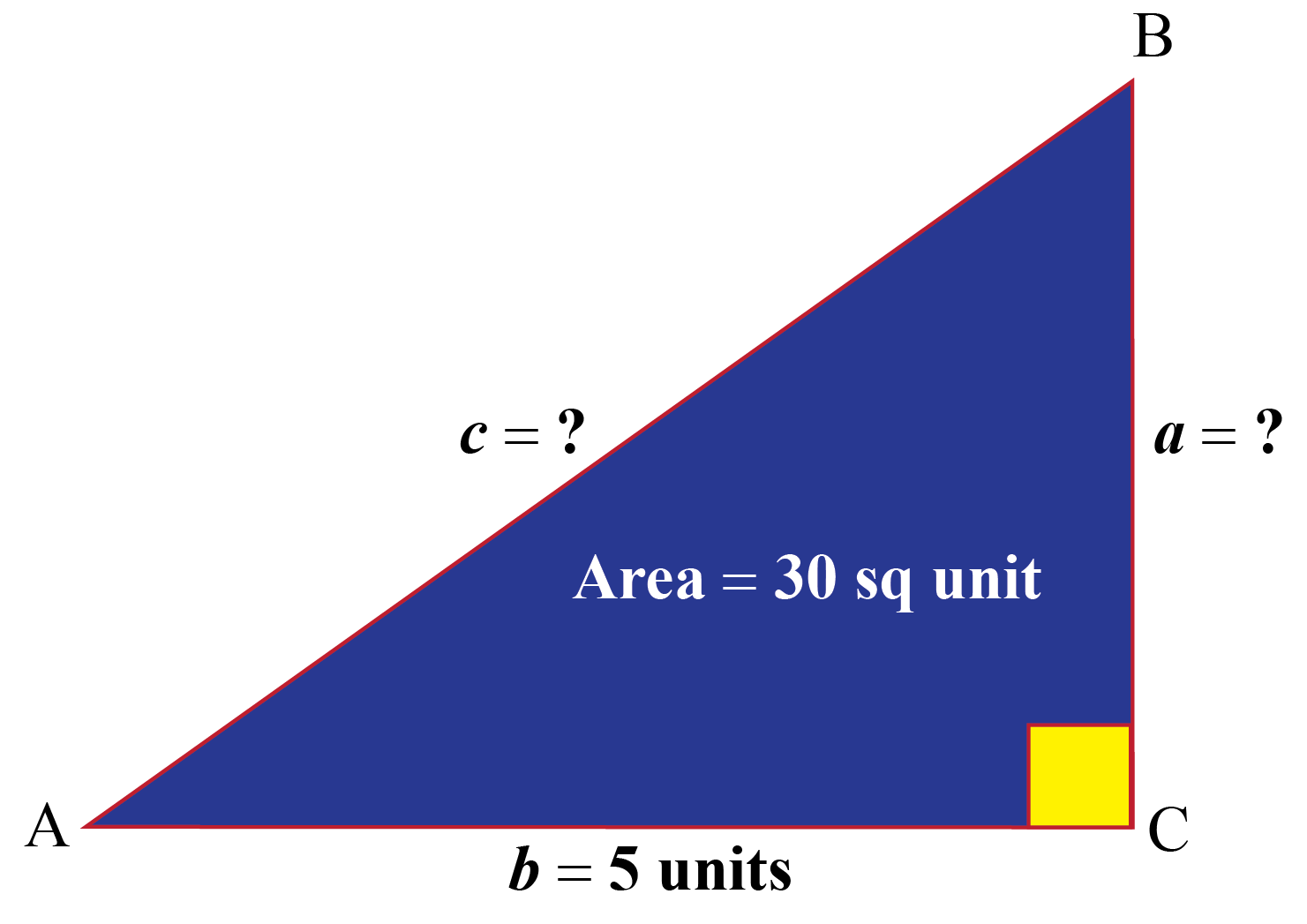Isabella knows area of triangle = \dfrac{1}{2} \times b \times a.

Hence,

$$30 = \dfrac{1}{2} \times 5 \times a$$

$$a = \dfrac{30 \times 2}{5} = 12$$

To find the third side "c" Pythogoras Theorem will be used which is given by the following formula,

$$a^2 + b^2 = c^2$$

$$12^2+5^2 = c^2$$

$$c^2 = 144 + 25 = 169$$

$$c = \sqrt{169} = 13$$

Hence, c = 13 units.

## Solved Examples

 Example 1

Michelle's teacher asked her to find the third side of triangle, if the length of a and b was mentioned as 6 units and 10 units respectively and the value of angle A was 30. Help her visualize the triangle and do the triangle calculation to find C.

Solution

Michelle will draw the triangle as follows: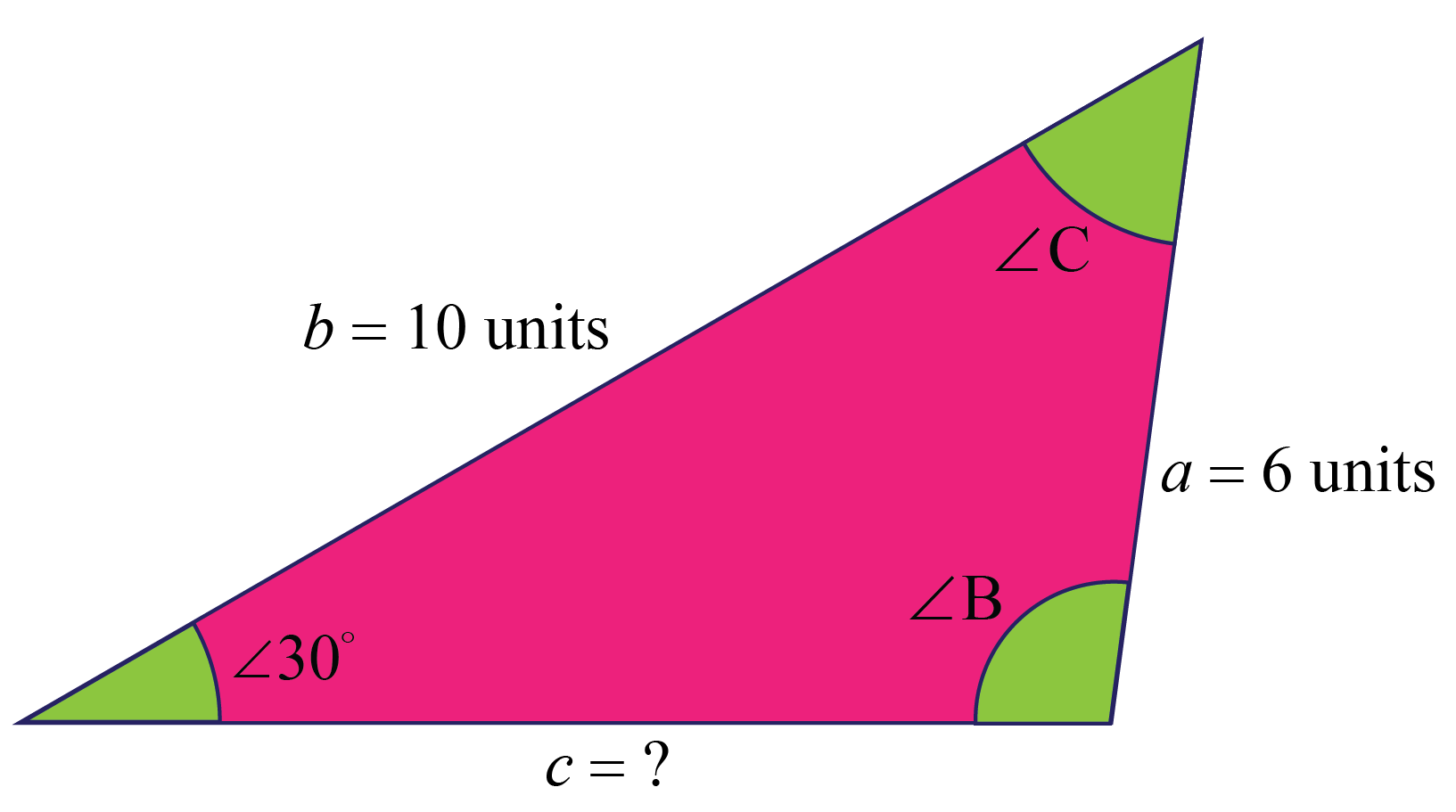To find the third side, Michelle uses the Law of Sines which is given as follows:

$$\dfrac{a}{\sin A} = \dfrac{b}{\sin B} = \dfrac{c}{\sin C}$$

Given the value of sides a and b, 6 units and 10 units respectively and A = 30. On applying the Law of Sines she gets,

$$\dfrac{6}{\sin 30} = \dfrac{10}{\sin B}$$

Hence, $$\sin B = \dfrac{10 \times \sin 30}{6}$$

$$\sin B = \dfrac{5}{6}$$

As, $$\sin 30 = \dfrac{1}{2}$$

B = $$\sin^{ - 1} 0.83$$

B =  56.09

Sum of all angles in a triangle is 180 .

Hence, A + B + C = 180

30 + 56.09 + C = 180

C = 180 - (30 + 56.09) = 93.91∘

Hence, by the Law of Sines,

$$\dfrac{10}{\sin 56.09} = \dfrac{c}{\sin 93.91}$$

c = $$\dfrac{10 \times \sin 93.91}{\sin 56.09}$$

c = 12.02 units

 $$\therefore$$ Michelle found length of third side is 12.02 units.
 Example 2

How will Leonard do the triangle calculation to find hypotenuse of a right angled triangle if the area of triangle is 84 unitand the perpendicular is 24 units?

Solution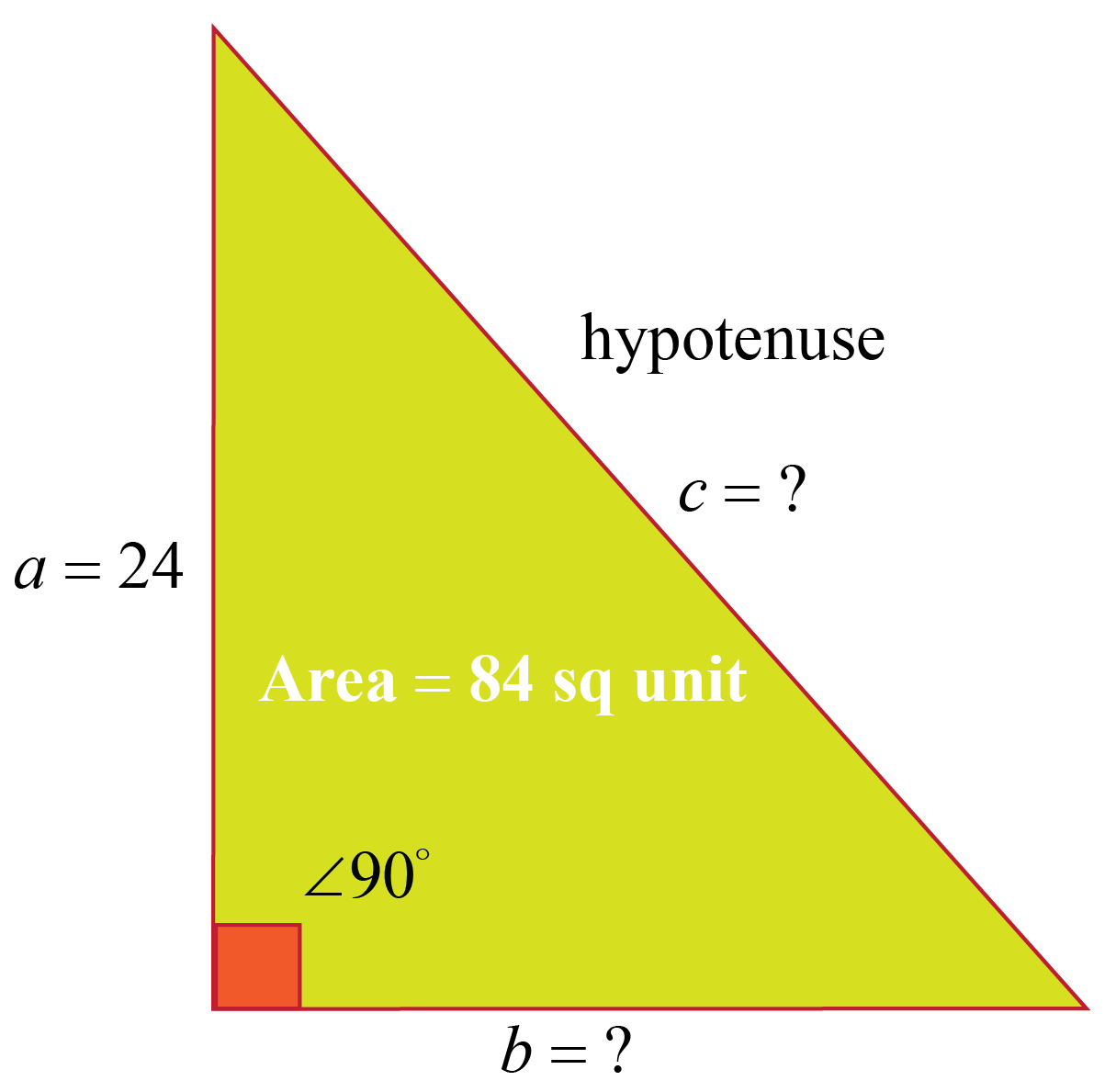Leonard knows area of triangle = $$\dfrac{1}{2} \times b \times a$$.

Hence,

$$84 = \dfrac{1}{2} \times b \times 24$$

$$b = \dfrac{84 \times 2}{24} = 7$$

To find the third side c Pythogoras Theorem will be used which is given by the following formula,

$$a^2 + b^2 = c^2$$

$$24^2 + 7^2 = c^2$$
$$c^2 = 576 + 49 = 625$$

$$c = \sqrt{625} = 25$$ units

 $$\therefore$$ Leonard found the length of third side to be 25 units.
 Example 3

How will Jenny prove that when the Law of Cosines is applied to the right angled triangle, it'll result in formula of Pythagorean Theorem?

Solution

Let's say Jenny assumes angle C  = 90∘

The Law of Cosines for angle C will be written as,

$$\cos C = \dfrac{a^2+b^2-c^2}{2ab}$$

As, cos 90 = 0

Applying in the above formula she will get,

$$\dfrac{a^2+b^2-c^2}{2ab} = 0$$

On cross multiplying 2ab she'll get,

$$a^2+b^2-c^2 = 0$$

This final equation is the formula of Pythagorean Theorem.

 Hence, the final equation obtained is $$a^2+b^2 = c^2$$.

## Practice Questions

Here are a few activities for you to practice.

## Conclusion

We hope you enjoyed learning about triangle calculation to find C with the simulations and practice questions. Now, you will be able to easily solve problems on triangle calculation to find H, triangle area calculator and right angled triangle calculation.

You can also simplify this topic with our Math Experts in Cuemath’s LIVE and interactive online classes. Book a FREE trial class today!

## 1. How do you find third side of a triangle?

We can find the third side of a triangle using any of the laws, either the Law of Sines or Law of Cosines can be used.

## 2. How do you solve special triangles?

The special triangle is a right-angled triangle. We can solve special triangle using the Pythagoras Theorem which helps in finding the third side "c" using the formula $$a^2 + b^2 = c^2$$.

## 3. How do you find the third side of an isosceles triangle?

We can divide the triangle into two halves. It can be treated as two right triangles and the Pythagorean Theorem can be applied on them to find the third sides.

More Important Topics
Numbers
Algebra
Geometry
Measurement
Money
Data
Trigonometry
Calculus
More Important Topics
Numbers
Algebra
Geometry
Measurement
Money
Data
Trigonometry
Calculus